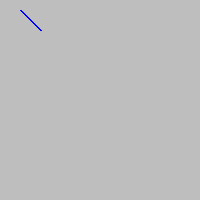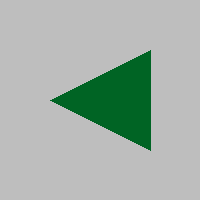# Obeleži sve kategorije koje odgovaraju problemu

### Još detalja - opišite nam problem

Uspešno ste prijavili problem!
Status problema i sve dodatne informacije možete pratiti klikom na link.
Nažalost nismo trenutno u mogućnosti da obradimo vaš zahtev.
Molimo vas da pokušate kasnije.

# Drawing straight lines and polygons¶

The functions for drawing straight lines and polygons are similar to the functions for drawing rectangles, ellipses, and circles, which we have already learned. The parameters canvas, color and thickness are used here as well, with the same meaning as before. We will explain new parameters as we come across them.

We will repeat the “empty” program here, which only handles the PyGame library and the drawing window (and does not draw anything by itself), in case you want to try something out quickly.

## Drawing a line¶

To draw a line we use the function pg.draw.line, with or without the thickness parameter.

pg.draw.line(canvas, color, point1, point2, thickness)
pg.draw.line(canvas, color, point1, point2)

• The parameters point1, point2 are points on the screen that represent the end points of the line segment. Again, a point is specified as a tuple or a list of 2 elements. The elements of this tuple or list are the coordinates of the point in the window in which we draw.

• With this function, omission of thickness has a different meaning than in other functions, which is to use the default line thickness of 1 pixel.

For example, the command:

draws a blue line 2 pixels wide from point $$(20, 10)$$ to point $$(40, 30)$$.## Drawing a polygon¶

To draw a polygon we use the function pg.draw.polygon, which also has two forms:

pg.draw.polygon(canvas, color, point_list, thickness)
pg.draw.polygon(canvas, color, point_list)

• The parameter point_list represents a list of vertices of the polygon we are drawing. For example [(50, 250), (150, 150), (250, 250)] is a list of 3 points.

• Here again, we use the form without the thickness parameter when we want the polygon to be the filled with the specified color (if we specify a width, a polygonal line of that width will be drawn).

For example, the following statement draws a triangle colored with $$(0, 100, 36)$$ color. The vertices of the triangle are $$(50, 100)$$, $$(150, 150)$$ and $$(150, 50)$$.In addition to these listed and described functions, there are other drawing functions in the pg.draw module, but we will not deal with them here. If you are interested in learning more about these functions, you can find more complete information for example at https://www.pygame.org/docs/ref/draw.html

## Drawing functions - questions¶

        Q-33: Q-32: In what order are these arguments given in a call of the pg.draw.line functioncanvas
color
first point coordinates
second point coordinates
thickness


Q-34: We want to draw a triangle. In what form can point coordinates be specified?

• pg.draw.polygon(canvas, color, [(0, 0), (50, 100), (100, 0)])
• Correct
• pg.draw.polygon(canvas, color, (0, 0), (50, 100), (100, 0))
• Try again
• pg.draw.polygon(canvas, color, (0, 0, 50, 100, 100, 0))
• Try again
• pg.draw.polygon(canvas, color, [0, 0, 50, 100, 100, 0])
• Try again

Q-35: Which of the following polygons cannot be drawn with multiple calls of the pg.draw.line function?

• pg.draw.polygon(canvas, color, [(0, 0), (50, 100), (100, 0)], 7)
• Correct
• pg.draw.polygon(canvas, color, [(0, 0), (0, 50), (50, 50), (50, 0)])
• Try again
• pg.draw.polygon(canvas, color, [(0, 0), (50, 100), (100, 0)])
• Try again
• pg.draw.polygon(canvas, color, [(0, 0), (0, 50), (50, 50), (50, 0)], 4)
• Correct
Pair the drawing statements and the shapes that they draw. Try again!
• Line segment
• pg.draw.line
• Polygon
• pg.draw.polygon
• Rectangle
• pg.draw.rect
• Circle
• pg.draw.circle
        Q-37: Q-36: Sort according to the typical order of arguments in the drawing functions:canvas
color
coordinates
thickness


Q-38: When drawing which of the following shapes are the coordinates given in the form of a list of ordered pairs?

• Circle
• Try again
• Ellipse
• Try again
• Polygon
• Correct
• Line segment
• Try again
• Square
• Try again

## Drawing by instructions¶

####Scarecrow: Draw a scarecrow on a white background. It consists of the following parts:

• head: a black circle, 6 pixels wide, centered at point (150, 70), of radius 50

• body: a straight black line, 6 pixels wide, from point (150, 120) to point (150, 300)

• arms: a straight black line, 6 pixels wide, from point (80, 170) to point (220, 170)

• left leg: a straight black line, 6 pixels wide, from point (150, 300) to point (90, 480)

• right leg: a straight black line, 6 pixels wide, from point (150, 300) to point (210, 480)

####Tree: Draw a tree on a white background. It consists of the following parts:

• trunk: a rectangle filled with color (97, 26, 9), size 40 x 50, with top left vertex at point (130, 250)

• upper part of the treetop: a triangle filled with color (0, 100, 36), with vertices (50, 250), (150, 150) and (250, 250)

• middle part of the treetop: a triangle filled with color (0, 100, 36), with vertices (75, 200), (150, 100) и (225, 200)

• bottom part of the treetop: a triangle filled with color (0, 100, 36), with vertices (100, 150), (150, 50) и (200, 150)

## Surprise drawings¶

To see the drawing in the tasks that follow, you need to write the right statements and run your program.

####surprise 1 - connect the dots: The vertices of a polygon are given. Draw that polygon filled with “khaki” color on a “darkgreen” background.

####surprise 2:

Using “limegreen” color, draw:

• A filled ellipse inscribed in a rectangle whose top left vertex is at (75, 100), its width is 150, and its height is 180;

• A line of width 6, from point (130, 110) to point (120, 20);

• Another line of width 6, from point (170, 110) to point (180, 20);

• A filled circle of radius 10 pixels, centered at point (120, 20);

• A filled circle of radius 10 pixels, centered at point (180, 20);

Using black color draw two more filled ellipses:

• one inscribed in a rectangle whose top left vertex is at point (110, 140), its width is 30, and its height is 50;

• and one inscribed in a rectangle whose top left vertex is at point (160, 140), its width is 30, and its height is 50;